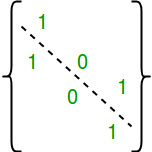Open in App
Not now

# Number of Symmetric Relations on a Set

• Difficulty Level : Medium
• Last Updated : 19 May, 2022

Given a number n, find out the number of Symmetric Relations on a set of first n natural numbers {1, 2, ..n}.

Examples:

```Input  : n = 2
Output : 8

Given set is {1, 2}. Below are all symmetric relation.
{}
{(1, 1)},
{(2, 2)},
{(1, 1), (2, 2)},
{(1, 2), (2, 1)}
{(1, 1), (1, 2), (2, 1)},
{(2, 2), (1, 2), (2, 1)},
{(1, 1), (2, 2), (2, 1), (1, 2)}

Input  : n = 3
Output : 64```

A Relation ‘R’ on Set A is said be Symmetric if xRy then yRx for every x, y ∈ A
or if (x, y) ∈ R, then (y, x) ∈ R for every x, y?A

Total number of symmetric relations is 2n(n+1)/2.
How does this formula work?
A relation R is symmetric if the value of every cell (i, j) is same as that cell (j, i). The diagonals can have any value.There are n diagonal values, total possible combination of diagonal values = 2n
There are n2 – n non-diagonal values. We can only choose different value for half of them, because when we choose a value for cell (i, j), cell (j, i) gets same value.
So combination of non-diagonal values = 2(n2 – n)/2
Overall combination = 2n * 2(n2 – n)/2 = 2n(n+1)/2

## C++

 `// C++ program to count total symmetric relations``// on a set of natural numbers.``#include ` `// function find the square of n``unsigned ``int` `countSymmetric(unsigned ``int` `n)``{``    ``// Base case``    ``if` `(n == 0)``        ``return` `1;` `   ``// Return 2^(n(n + 1)/2)``   ``return` `1 << ((n * (n + 1))/2);``}` `// Driver code``int` `main()``{``    ``unsigned ``int` `n = 3;` `    ``printf``(``"%u"``, countSymmetric(n));``    ``return` `0;``}`

## Java

 `// Java program to count total symmetric``// relations on a set of natural numbers.``import` `java.io.*;``import` `java.util.*;` `class` `GFG {` `    ``// function find the square of n``    ``static` `int` `countSymmetric(``int` `n)``    ``{``        ``// Base case``        ``if` `(n == ``0``)``            ``return` `1``;``    ` `    ``// Return 2^(n(n + 1)/2)``    ``return` `1` `<< ((n * (n + ``1``)) / ``2``);``    ``}` `    ``// Driver code``    ``public` `static` `void` `main (String[] args)``    ``{``        ``int` `n = ``3``;``        ``System.out.println(countSymmetric(n));``    ``}``}`  `// This code is contributed by Nikita Tiwari.`

## Python3

 `# Python 3 program to count``# total symmetric relations``# on a set of natural numbers.` `# function find the square of n``def` `countSymmetric(n) :``    ``# Base case``    ``if` `(n ``=``=` `0``) :``        ``return` `1`` ` `    ``# Return 2^(n(n + 1)/2)``    ``return` `(``1` `<< ((n ``*` `(n ``+` `1``))``/``/``2``))` ` ` `# Driver code` `n ``=` `3``print``(countSymmetric(n))` `# This code is contributed``# by Nikita Tiwari.`

## C#

 `// C# program to count total symmetric``// relations on a set of natural numbers.``using` `System;` `class` `GFG {` `    ``// function find the square of n``    ``static` `int` `countSymmetric(``int` `n)``    ``{``        ``// Base case``        ``if` `(n == 0)``            ``return` `1;``    ` `    ``// Return 2^(n(n + 1)/2)``    ``return` `1 << ((n * (n + 1)) / 2);``    ``}` `    ``// Driver code``    ``public` `static` `void` `Main ()``    ``{``        ``int` `n = 3;``        ``Console.WriteLine(countSymmetric(n));``    ``}``}`  `// This code is contributed by vt_m.`

## PHP

 ``

## Javascript

 ``

Output

`64`

My Personal Notes arrow_drop_up